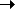Package net.flashpunk.tweens.motion Class public class QuadPath Inheritance QuadPathMotionTweenObject

A series of points which will determine a path from the beginning point to the end poing using quadratic curves.

Public Properties
PropertyDefined Byactive : Boolean
If the tween should update.
Tweencomplete : Function
Tween completion callback.
Tweendelay : Number = 0
Length of time to wait before starting this tween.
Tweenobject : Object
Target object for the tween.
Motionpercent : Number
The completion percentage of the Tween.
Tween
pointCount : Number
[read-only] Amount of points on the path.scale : Number
[read-only] The current time scale of the Tween (after easer has been applied).
Tweenx : Number
Current x position of the Tween.
Motiony : Number
Current y position of the Tween.
Motion
Protected Properties
PropertyDefined By_object : Object
Motion_x : Number = 0
Motion_y : Number = 0
Motion
Public Methods
MethodDefined By

QuadPath(complete:Function = null, type:uint = 0)
Constructor.

addPoint(x:Number = 0, y:Number = 0):void
Adds the point to the path.cancel():void
Immediately stops the Tween and removes it from its Tweener without calling the complete callback.
Tween

getPoint(index:uint = 0):Point
Gets the point on the path.

setMotion(duration:Number, ease:Function = null):void
Starts moving along the path.

setMotionSpeed(speed:Number, ease:Function = null):void
Starts moving along the path at the speed.start():void
Starts the Tween, or restarts it if it's currently running.
Tweenupdate():void
Updates the Tween, called by World.
Tween
Public Constants
ConstantDefined ByLOOPING : uint = 1
[static] Looping Tween type, will restart immediately when it finishes.
TweenONESHOT : uint = 2
[static] Oneshot Tween type, will stop and remove itself from its core container when it finishes.
TweenPERSIST : uint = 0
[static] Persistent Tween type, will stop when it finishes.
Tween
Property Detail
 pointCount property
`pointCount:Number`  [read-only]

Amount of points on the path.

Implementation
`    public function get pointCount():Number`
Constructor Detail
`public function QuadPath(complete:Function = null, type:uint = 0)`

Constructor.

Parameters
 `complete:Function` (default = `null`) — Optional completion callback. `type:uint` (default = `0`) — Tween type.
Method Detail
` public function addPoint(x:Number = 0, y:Number = 0):void`

Adds the point to the path.

Parameters

 `x:Number` (default = `0`) — X position. `y:Number` (default = `0`) — Y position.

 getPoint () method
` public function getPoint(index:uint = 0):Point`

Gets the point on the path.

Parameters

 `index:uint` (default = `0`) — Index of the point.

Returns
 `Point` — The Point object.
 setMotion () method
` public function setMotion(duration:Number, ease:Function = null):void`

Starts moving along the path.

Parameters

 `duration:Number` — Duration of the movement. `ease:Function` (default = `null`) — Optional easer function.

 setMotionSpeed () method
` public function setMotionSpeed(speed:Number, ease:Function = null):void`

Starts moving along the path at the speed.

Parameters

 `speed:Number` — Speed of the movement. `ease:Function` (default = `null`) — Optional easer function.